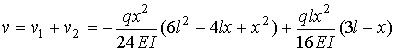Beam deflection by superposition of solutions

# Basic idea:

Since the beam deflection equation is linear, any combination of solutions to this equation is itself a solution to the beam equation. By adding known solutions of the beam equation together, one can construct new solutions to the beam equation. In this way one can construct a variety of solutions to fit a large number of boundary conditions. Tables of solutions to the beam deflection equation can be found in most textbooks.

The key issue in construction of solutions using the method of superposition is that one select a set of knows solutions that in combination can satisfy the boundary conditions of the problem under consideration. Clearly this method is only useful when we can find solutions that when combined are capable of satisfying the boundary conditions of the problem under consideration.

# Example:

Consider the propped up cantilever beam show in the figure.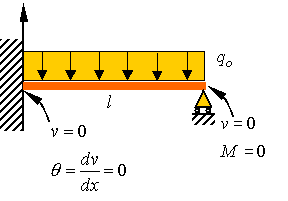The boundary conditions for this problem are indicated on the figure above. One can consider this problem as the summation of the two problems shown in the figureClearly both solutions satisfy the zero displacement and zero slop condition at the left side. Both solution satisfy the zero moment condition on the right side. We simply need to make the combined solution satisfy the zero displacement solution at the right tend of the beam. This can be done by selecting the reaction force R in the second solution large enough so that the combined solution does has zero displacement at the right side of the bar. For the first solution the deflection downward of the right end is given by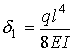For the second solution the deflection downward is given by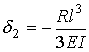To satisfy the zero displacement condition at the right end in the original problem, we set the total displacement equal to zero and solve for the support reaction R. That is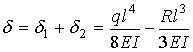## This yields the unknown reaction force at the right support as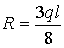The solutions for the upward displacement of for each solution is given as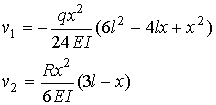Combining the two solutions and substituting for the support reaction yields the solution to the propped up cantilever as